Lead Acid batteries typically tend to be cheaper than LifePO4 batteries. This cheap cost comes with some considerations however, as lead acid batteries are heavier, larger, less efficient and have fewer life cycles. Below, we've broken down why that smaller upfront cost can end up actually having you spend more per kwh. Remember that no battery is 100% efficient and factors such as C rating, Depth of discharge, life cycles, and temperature all play a role in the cost of a unit. Below is an in depth breakdown and cost comparison of Lead Acid vs LifePO4 batteries.

Calculating Actual Energy at different C ratings

C rating: The ratio at which you are drawing kW compared to the kWh rating. A 4 kWh battery being discharged at 2 kW would have a 0.5C discharge.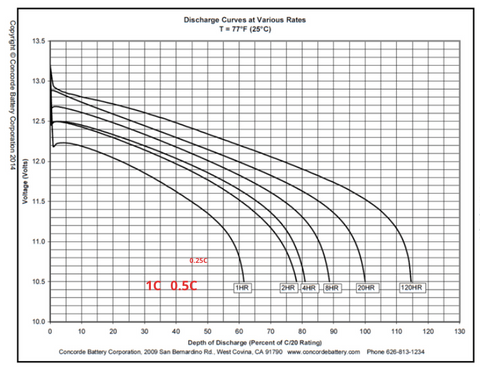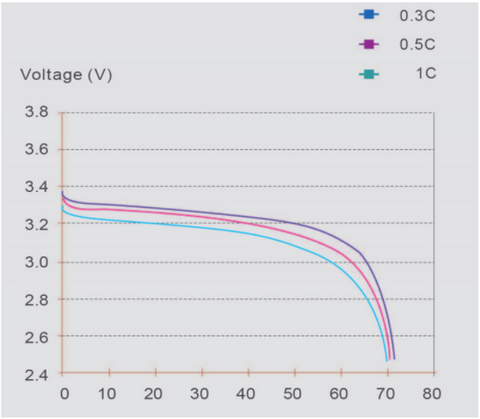Life Cycles & Depth of Discharge Definitions

Depth of discharge (DOD): The percentage of the total battery capacity consumed while discharging. Example: Discharging a 100Ah battery by 60Ah is a 60% DOD.

Life cycles: The number of times a battery can be charged and discharged at that DOD percentage.

Calculate Usable energy based on your expected life cycles

A 4kWh battery with a 2 kW load.

Our C value = 2/4 Therefore 0.5C

Using the graph from the previous slide, our real capacity at 0.5C is 77% of 4kWh, so ~3kWh.

In order to get 1000 Cycles, we would have to discharge the battery to only 50% based on the cycle graph.

Result:

A 4kWh deep cycle battery that will provide 1000 cycles can only provide you with 1.5kWh!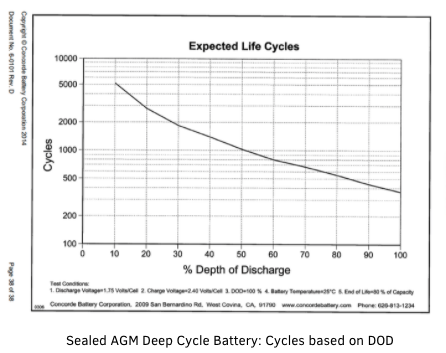Lithium batteries life cycles are based on 3 things:

• Depth of Discharge (DOD)
• Temperature
• C rating

LiFePO4 battery under the same conditions as previous example:

• 50% DOD
• 0.5C
• 25 Deg C

Results:

Up to 7500 cycles at 2kWh of usable energy (from original 4kWh)

Or

3500 cycles using the full 4kWh.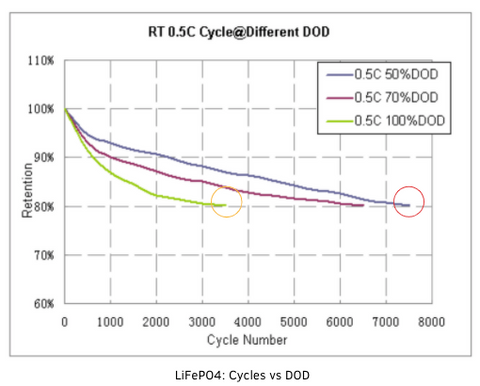Cost Analysis

When determining cost, remember you're paying for each cycle.

Building on our example of the 4kWh battery with a 2kW load, 50% DOD and 80% remaining energy after the rated cycles have been used.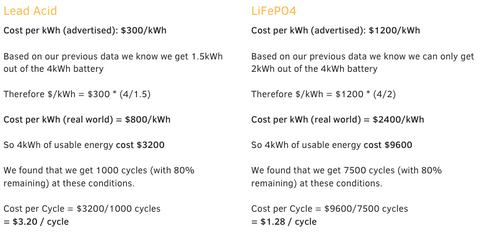Cost per Cycle RESULTS: